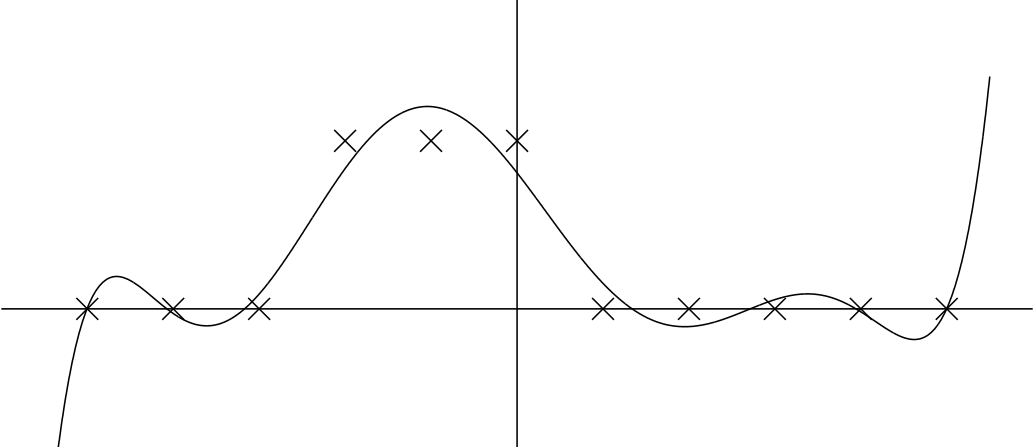# Math 614 Numerical Linear Algebra

## Fall 2021, UAF

instructor details
Ed Bueler time:  MWF 10:30--11:30am
Chapman 306C room:  Chapman 206  107 (or web-based)
elbueler@alaska.edu in person section:   F01, crn 74997
bueler.github.io online section:   FXA, crn 75737

The course is for graduate students and advanced undergraduates with a need for computation in any field: statistics, computer science, geophysics, engineering, biology, ... mathematics too! Students will discover a big picture that they missed in undergraduate linear algebra (and graduate algebra). Besides the mathematical concepts, a key outcome of this course is familiarity with core computational techniques on vectors (i.e. high-dimensional data).

In fact, linear algebra is everywhere in the application of mathematics. Huge linear systems are solved routinely on computers, and the science and engineering worlds depend on it. This course will describe how matrices and vectors can be handled on computers in a stable, fast, and accurate manner. We will place these topics in their correct mathematical context, finite-dimensional vector spaces. The emphasis will be on geometric, algorithmic, and practical understanding rather than algebraic proof.We will cover these tools and topics:

• singular value decomposition (SVD = principal component analysis)
• conditioning of problems and stability of algorithms
• Gauss elimination and its variants for structured matrices
• over- and under-determined systems
• least squares methods
• power and QR methods for eigenvalues
• Krylov subspace methods, iterative linear algebraWe will use Matlab (or Python or Julia) in lectures and homework assignments. Most homework will include computational examples. Introductory Matlab examples at the beginning of the course will bring students up to speed with that tool. And there will be some proofs; they will make up about 30% of the homework.

Prerequisites:  Officially,   MATH F314 Linear Algebra. Recommended: MATH F401 Intro Real Analysis or MATH F432 Intro Partial Differential Equations.   Informally, this means an undergraduate course in linear algebra plus some exposure to calculus/analysis concepts beyond the standard freshman/sophomore calculus sequence. And in practice, permission of instructor.

Textbook: Trefethen and Bau, Numerical Linear Algebra, SIAM Press, 1997. (rent at UAF Bookstore; purchase \$70 at bookstore.siam.org or \$50 for SIAM members)